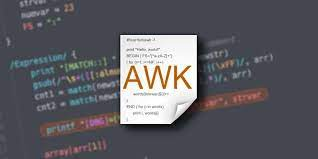# How to Use Shell Variables in Awk Script

Awk is a powerful Linux command to work with files & strings. But sometimes you may need to use shell scripts within your awk commands. This can be tricky since shell variables are prefixed with \$ sign, which has special but different meaning in awk commands too. If you directly use shell variables in awk command, it will interpret the variable names as per awk’s syntax and not as shell variables. This will give you undesirable results. In this article, we will learn several ways to use shell variables in Awk script.

## How to Use Shell Variables in Awk Script

We will look at the different ways to use shell script variables in awk script.

## 1. Using -v option

In this case, you need to mention your shell variable after awk command, -v option and a space, but before BEGIN statement. Here is an example where we use shell variable \$shell_var in awk command by assigning it to awk variable var.

```shell_var="line one\nline two"
awk -v var="\$shell_var" 'BEGIN {print var}'
line one
line two```

If you want to mention multiple variables, use -v option for each variable as shown below. In the following example, we use two shell variables var1 and var2.

`awk -v a="\$var1" -v b="\$var2" 'BEGIN {print a,b}'`

This method is useful if your awk statement contains BEGIN code.

## 2. Without -v option

If your awk command does not contain BEGIN code, you can easily mention these variables along with their values, inline. Here is an example.

```variable="line one\nline two"
echo "input data" | awk '{print var}' var="\${variable}"
or
awk '{print var}' var="\${variable}" file```

In both the above commands, we use shell variable \$variable in our awk command by assigning it to var awk variable.

You can also use multiple shell variables as shown below, where we assign shell variables var1 and var2 to a and b respectively.

`awk '{print a,b,\$0}' a="\$var1" b="\$var2" file`

## 3. Using String

You can also pass shell variables as strings within double quotes, using pipe operator ‘|’ or using a here string(<<<) operator. Here are two examples using them.

```awk '{print \$0}' <<< "\$variable"
test```

Using pipe operator

`printf '%s' "\$variable" | awk '{print \$0}'`